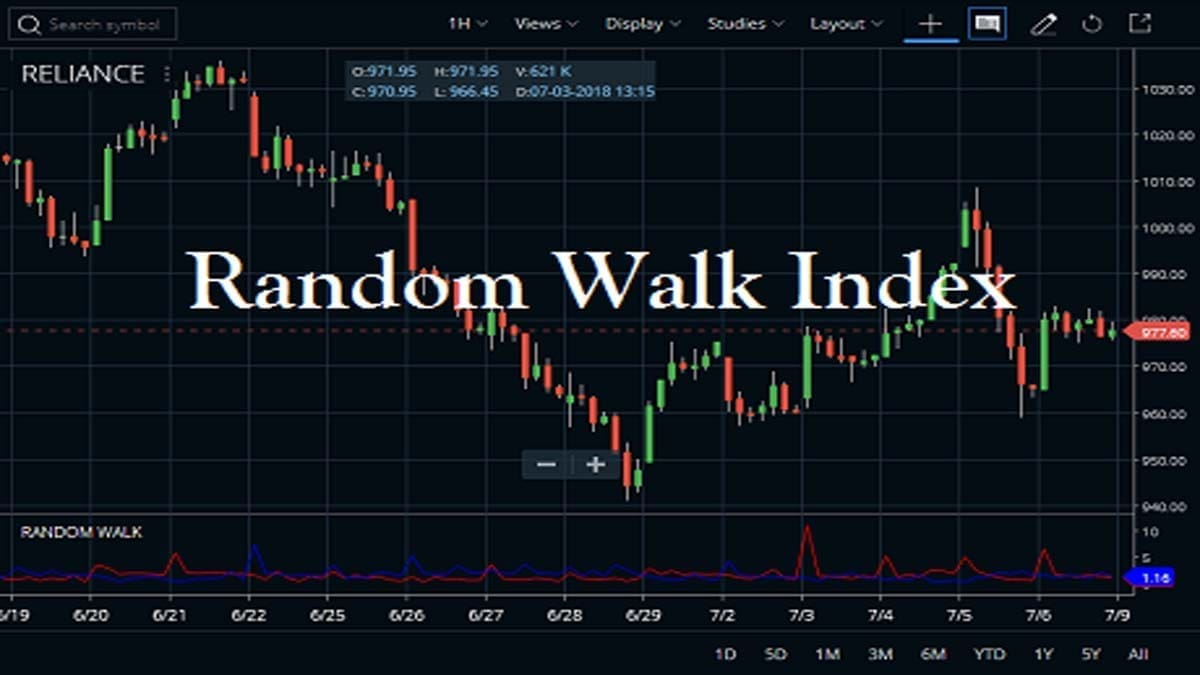Home » Blog » Trading » Technical Indicators » Random Walk Index Indicator Set up, Calculation, Usage

# Random Walk Index Indicator Set up, Calculation, UsageRandom Walk Index Indicator is a technical indicator that determines if a security is trending or in a random trading range. By measuring price ranges over N the indicator can identify a strong uptrend or downtrend. The greater the range suggests a stronger trend. Traders can also use the indicator to generate buy-sell signals. So, let’s begin from the very basic.

## What is the Random Walk Index Indicator?

Michael Poulos introduced the Random Walk Index in Technical Analysis of Stocks and Commodities magazine. The main purpose of the indicator is to determine whether the price is significantly trending or whether it just makes random movement ups and down. Though it was developed specifically for the stock market, traders can use this for all asset types and all time frames.

The more random the asset price movement, the more the Rwi indicator fluctuates.  The short-term (2 to 7 periods) Rwi is an overbought or oversold indicator, and the long-term (8 to 64 periods) RWI is a trend indicator.  Financial security is trending higher when the RWI of the highs is greater than 1. And, on the other side, a downtrend is indicated if the Rwi of the lows is greater than 1.

## The formula of Random Walk Index Indicator

By using a certain mathematical principle, you can calculate uptrend and downtrend. As a result, you can determine if it’s random or statistically meaningful.

As the indicator measures both uptrend and downtrend, the calculation requires seperately.

High periods, or RWI High calculation, is:

The formula is High minus Low(n) divided by the average true range (ATR) multiplied by the square root of n.

Therefore, if you are calculating the RWI High of the last five days, here take the high from today and minus the low from the prior period and calculate RWI High. Then, calculate using today’s high minus the low two days before. Do these steps for each day going back five trading sessions.

Now, the RWI High value is the highest value of the last five days or for many periods (n) were chosen.

On the other side, RWI Low is calculated as follows:

Here, the formula is High(n) minus Low divided by the average true range (ATR) multiplied by the square root of n.

The process is similar to the approach above, except now you will use today’s low and the high from the previous period to create the first calculation. After that, use the high from two days ago. Do these steps for each of the n periods.

## What is the Usage Process of the Random Walk Index Indicator?

If traders want to use the Random Walk Index (RWI) indicator, then they can find it in the STUDIES section of Zerodha Kite. Also, this is available in the Kite mobile App. The default PERIOD is 14 and you can set the colors of the high and low. You can check how we attached the Random Walk Index indicator in Reliance Industries share price chart. This works on any charts like daily, weekly, monthly or intraday you can attach.

Now, come to another charting platform Upstox Pro. Just like Zerodha kite, open Upstox Pro. Then select any chart and type down the indicator name on the search box. Finally, click on apply.

## How Strategically can you use the Random Walk Index Indicator?

• Random Walk Index or RWI indicator is mainly a combination of two lines the red line and the green line. The red line denotes the strength of the downward movement. And on the other side, the green one denotes the strength of the upward movement.
• There are also multiple levels — horizontal lines marked as 1, 2 and 3. Once green and red lines fluctuate around ‘1’, most price moves are random. And, once either of the lines moves above ‘2’, the price action can be attributed to a trend.
• Now, if one of the lines crosses ‘3’ from below, a particularly strong trend is observed.
• Here, a trader can consider opening a BUY position once the green line is above 1.5. And, the red line is below 1 level.
• Reversely, open SELL position when the red line is above 1.5, and the green line is below 1 level. All moves below 1 are considered random price fluctuations.
• You can combine it with any other momentum or a volatility indicator. MA or Moving Average also works quite well. Hence, you can use RWI as a supportive tool that will confirm signals sent by other indicators. The second strategy will generally mean that you receive fewer signals but of higher advance quality.